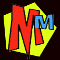# USA VISITORS! The MM books are nowavailable from FUN BOOKS or HORRIBLE BOOKS A Guide to the Maths in the Murderous Maths Books

## THE MATHS CONTENT OF THE INDIVIDUAL BOOKS

#### The first two books each contain a variety of little unconnected maths topics to create general interest. Many of the topics in these first two books are dealt with in much more detail in the further books. ###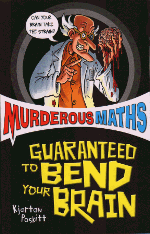Murderous Maths: Bend Your Brain A rough guide to the signs: + - x / % and powers Tessellation: covering surfaces with shapes. Roman Numerals, the development of the "10" and the place system. Shortcomings of calculators A brief explanation of prime numbers Time - how the year and day got divided, digital/analogue clocks A brief introduction to angles Introducing some real Mathematicians Magic squares - how to make your own Short cuts, rough mental arithmetic A card trick with algebra explanation Rounding off long numbers Symmetry - reflection and rotation ###Murderous Maths: Mash Your Mind Domino and Pentomino patterns and puzzles The difference between length, area and volume, also the difference between worlds of 1, 2 and 3 dimensions Measuring areas and volumes and basic rectangle/triangle formulas Speed, calculating and coverting m.p.h. to metres per sec etc. Mobius strips Pythagoras, right angled triangles, irrational numbers, pi Area and perimeter Bisecting angles in geometry Triangle numbers Simple topology networks "Mystery" number squares and how to make them. The first two books have now been edited together to produce a book suited to younger readers: The Brain-Bending Basics. Following the success of the first two books, a full series was planned covering the subject more comprehensively. The next two books explain all the basic maths you need to understand any other book in the series.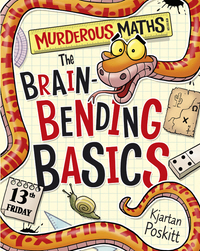###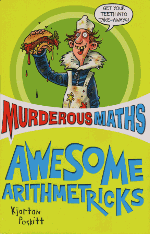The Awesome Arithmetricks Introduction to numbers, explanation of odd, even and negative numbers Signs - just about every sign in maths is explained fully. Place value (including decimal places) and rounding off Manipulating equations ADDITION: adding several big numbers at once, carrying over. SUBTRACTION: subtraction big numbers, working out change in shops. Time tables, also how to deal with e.g. 700 x 60. MULTIPLICATION: e.g. 12834 x 217 without a calculator. LONG DIVISION: leading to remainders, simple decimals and fractions Estimating answers to big sums ###The Mean and Vulgar Bits Introducing different fractions, converting improper and mixed fractions. Multiplying and dividing by vulgar fractions Explanation of primes, splitting numbers into prime factors Reducing fractions Highest Common Factors and Lowest Common Denominators Adding and subtracting different fractions Egyptian Fractions Comparing fractions Converting fractions to decimals, decimal place system Percentages: increase and decrease Averages: calculating means, also modes and medians explained The Awesome Arithmetricks and The Mean and Vulgar Bits have now been edited together to produce a book suited to younger readers: The Secrets of Sums. Having established the "basics" the rest of the books each follow a separate theme.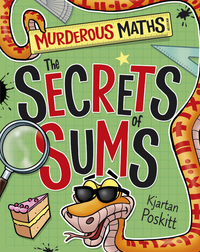###Desperate Measures Measuring lines - units and accuracy Old measuring systems and the development of metric The SI system and powers of ten. Shadow sticks and trundle wheels Names of different shapes Measuring areas and area formulas What weight really is Angles and how to measure them Measuring volume, Archimedes Principle Density Measuring time and how the modern calendar developed. Units to measure other things ###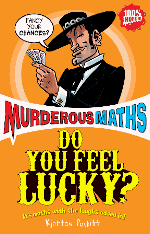Do You Feel Lucky? This book deals with probability starting with the very basics, but following the subject well past the confines of the usual school curriculum. Calculating chances on anything including tossing coins, throwing dice, dealing card hands and sharing birthdays Tree diagrams Mutually Exclusive and Independent chances Pascal's Triangle Permutations and Combinations Sampling ###Savage Shapes This is the pure geometry book which has no sums! Signs in geometric diagrams Loci explained Constructions: perpendicular bisectors, dropping perpendiculars, bisecting angles Triangles: different types, similar, congruent, equal areas Polygons: regular, irregular, angle sizes and construction. Tessellations and Penrose Tiles Simple origami Circles: chord, tangent and angle theorems. Regular solids, Euler's formula Ellipses, how to construct, eccentricity Geometric proof of Pythagoras' Theorem ###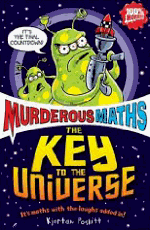The Key to the Universe This book is more of a "feature" book rather than an instruction book. It's packed with facts on numbers that have strange properites. Fibonacci Series and the Golden Ratio Properties of Square, Triangle, Cube, Centred Hexagon and Tetrahedral numbers The "difference of two squares" explained Superstitions arising from numbers Prime numbers - history including Mersenne primes Tests to see if a number will divide by anything between 2 and 13, also 19. Finger multiplication Binary and base 8 number systems Perfect numbers Irrational, transcendental and imaginary numbers explained The strange properties of infinity ###The Phantom X This book has just about everything you ever need to know about algebra. How letters are used for "unknowns" Creating and solving simple linear equations Brackets, factorising and expanding (or multiplying out) Simplifying expressions Solving quadratics and the quadratic formula "Think of a number" tricks Difference of two squares Coefficients of (a-b)n Linear graphs, including co-ordinates, gradients, y intercept, solving simple simultaneous equations Other non-linear function graphs including parabolas Solving simultaneous equations by substitution or elimination The perils of dividing by zero! ###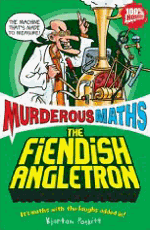The Fiendish Angletron Here is everything needed to understand Trigonometry including ratios and geometry How scales and ratios work on maps and diagrams (plus other ratio examples) How to do accurate constructions using a protractor and compasses Explanations of the SIN, COS and TAN ratios in right angled triangles How to use a calculator to get trig values (both normal and inverse) The sin and cosine formulas for non-right-angled triangles Measuring far away distances with triangulation Measurements in space (including explanations of parallax angles and parsecs) Sin/cos/tan relationships Drawing a sin wave, and what they sound like Bearings - finding positions at sea and plotting a course ###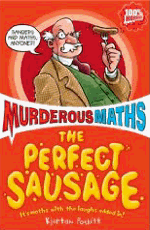The Perfect Sausage An indexed reference book containing about 180 formulas covering just about every topic in maths with explanations. Although presented in the usual Murderous Maths style, it will give additional support for anyone taking exams up to the age 16 level (including GCSE). Formulas include: All common areas and volumes, and many unusual ones including ellipsoids and toruses. Number formulas (e.g. triangle, hexagonal) Speed, acceleration, stopping times and distance Force, gravity, range of projectiles Money - percentages, simple and compound interest Day of the Week Algorithm Permutations and Combinations Many miscellaneous formulas See the full index ###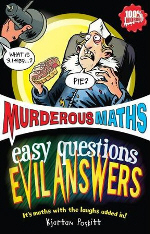Easy Questions Evil Answers A wide range of strange and bizarre bits of maths to satisfy the most curious minds! Presented in a question and answer format, lots of key maths topics are demonstrated in use including: Formulas Working out square roots by hand π Pythagoras Paradoxes Problem solving Metric prefixes A guide to massive numbers An introduction to vectors To get a better idea of the complete contents please see the list of questions. ###Professor Fiendish's Brainbenders Over 100 different challenges, many of which are maths based. Puzzle types include mazes logic coin problems number crosswords shape cutting/rearranging number squares ###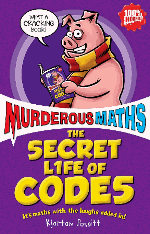The Secret Life of CODES Everything from basic substitution codes up to the RSA code used for sending credit card details over the internet! patterns logic and deduction prime numbers high powers MOD function ### The Murderous Maths of Everything The first large format full-colour MM book covers a huge range of topics with the focus on FUN! Prime numbers Sieve of Eratosthenes Pythagoras' Theorem Triangle numbers Square numbers The International Date Line Geometry (The three problems of antiquity) Geometric constructions Topology Complex Mobius strips Curves (including the conic sections and the cycloid) Golomb Rulers 4 dimensional 0's and X's (or "Tic Tac Toe") The Golden Ratio Fibonacci series Equiangular Spirals Musical ratios Theorems (including Ham Sandwich Theorem and Fixed Point Theorem) Probability (cards, dice and other games) Pascal's Triangle Sierpinski Triangle Chess board curiosities Light years The size and distances of the moon and the planets How planets orbit The sizes of stars and the shape of the galaxy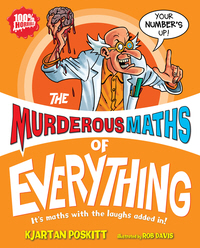Teaching math to young homeschool students can be fun when applied to the activities of daily home life. Renovations and upgrades to a residence offer multiple opportunities to teach practical money and measuring skills. Students will be able to tackle questions like what is the cost of a home elevator? Or how much weight can a home elevator hold?

##### WHAT THE PAPERS SAY Read the Times Educational Supplement and Primary Times reviews of "Diabolical Brainbenders"
The Complete Book Index

The Online Bookshop

The Full Site Contents at a Glance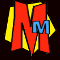Murderous Maths Main Index Page